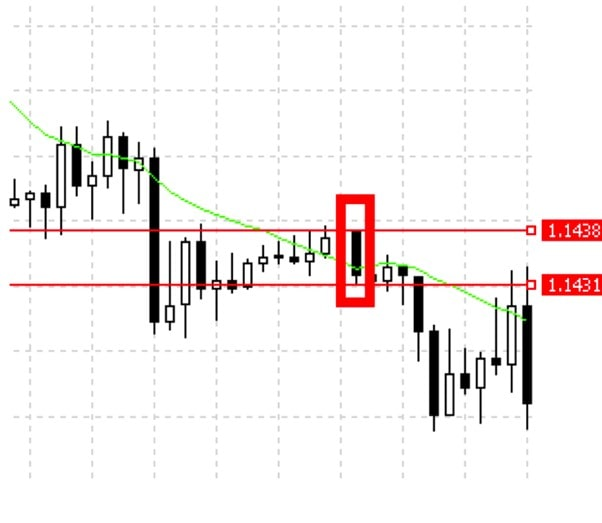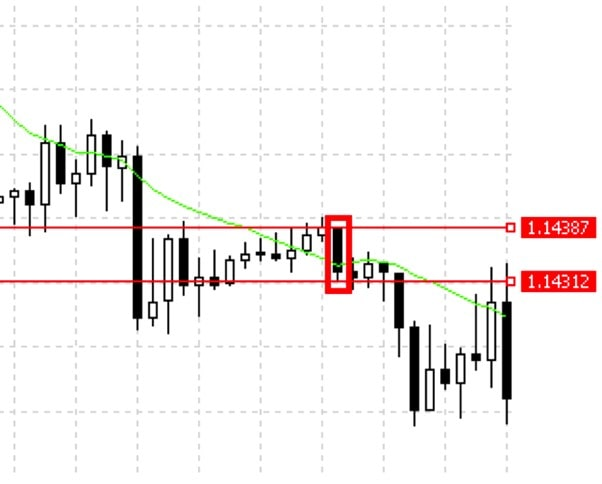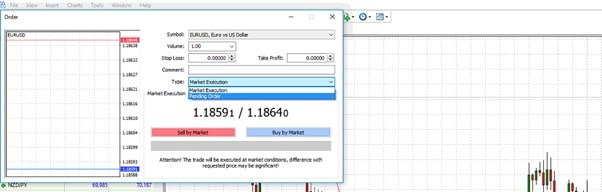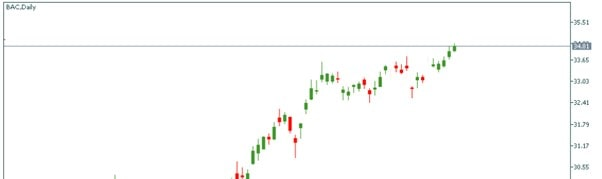Risk Warning: CFDs are complex instruments and come with a high risk of losing money rapidly due to leverage. 85.9% of retail investor accounts lose money when trading CFDs with this provider. You should consider whether you understand how CFDs work and whether you can afford to take the high risk of losing your money. Please click here to read our full Risk Warning.

85.9% of retail investor accounts lose money when trading CFDs with this provider.## Zero commission fees for trading any crypto CFD

• 0% commission fee
• 0% swap for overnight positions
• 0% commission for deposits

85.9% of retail investor accounts lose money

Available for retail clients on the Libertex Trading Platform# What Is a Pip in Trading?

Maybe you've been in the middle of watching YouTube, and out of the blue, an ad appears with a guy telling you how to make money in trading. The ad catches your attention, and you decide to hear him out. Then, just as it gets more interesting, the guy starts talking about 100 pips a day. The seemingly exciting ad suddenly confuses you.

If you started to wonder, "What's a pip? And why did he quantify his profits in pips?", don't worry. This article will expand your knowledge about pips in trading!

What is a pip? It's an abbreviation for "percentage point" and represents the smallest unit of change in a currency pair's value. For most currencies, especially the majors, a pip is the fourth decimal place in the two currencies' exchange rate. However, this decimal place may vary for some currency pairs. For example, in those that involve the Japanese yen, a pip is represented by the second decimal place.

What is a pip? A pip is an abbreviation for "percentage point," representing the smallest unit of change in a currency pair's value.

Suppose you're trading EUR/USD. You open a long position when the exchange rate is 1.2712, predicting that the price would increase. After a few minutes, the price moves to 1.2713, and you decide to close your trade. The price change here is 0.0001, which equals one pip.Pips reflect both your possible gains and losses. Say you open a long position when the price is 1.1438, as shown in the table below. You predict it will go up, but the price is, in fact, trading in the opposite direction. So you close the position when the exchange rate is 1.1431. How much have you lost? The entire change in the currency pair's value is 0.0007, which equals seven pips.It seems clear and easy until you notice that most trading platforms count price change in five or three decimals. What are these fifth and third figures?

## What Is a Pipette?

Many trading platforms use pips as their smallest units of measurement for the change in the currency pair's value. However, the need for more accuracy has led to the introduction of the pipette, which is 1/10 of a pip. In this case, a pipette is represented by the fifth decimal place. When the JPY is involved in the currency pair, a pipette stands for the third decimal place.Let's use the previous example, but this time with a broker platform that displays the value of pipettes.In this example, you open a long position when the exchange rate is 1.14387, expecting the price to rise. Unfortunately, that was not the case. Now you decide to close your trade at 1.14312, losing 0.00075, or 75 pipettes. Looking at this example, you'll appreciate the accuracy that pipettes provide.

Pipettes provide the trader with a higher degree of accuracy than pips.

In the previous example, the loss was seven pips. But now we get a clearer picture with the more granular unit of measurement: 75 pipettes (7.5 pips).## Points: Another Measurement

Now you know what a pip and pipette are. There's another important term that should be mentioned when talking about pips in trading: points. Let's take a look at an example in MetaTrader 4.

After you choose a pair to trade, you should place an order. All the parameters are set in a window that reflects the current bid and ask prices. We chose the EUR/USD pair. The ask price is 1.18640, and the bid price is 1.18591. The spread, the commission your broker takes for placing the order, is 4.9 pips.If you need to modify the trade parameters, you can do that easily, but you'll deal with points. Look at the image below. Levels are depicted in points. Here, points refer to the fifth decimal place. Thus, by changing the value by 10 points, you'll put your order just a pipette away.### The Importance of Pips in Trading

You use pips in trading to quantify how much you've made or lost on a particular trade. Also, expressing your profits or losses in currency amounts can be confusing and difficult to compare. That's because the number of dollars gained or lost depends on many factors. Additionally, pips serve as a unified counting system that all participants in the financial market understand.

## How to Calculate Pips in Trading

Now you know what a pip is and how to determine its size. That said, it's still unclear how a pip relates to possible profits.

### USD as a Quote Currency

We'll start with the easiest pip calculation method. Many trading accounts are denominated in the US dollar. The pip value doesn't change when the USD is listed second in a currency pair and the account is denominated in US dollars.

The pip value doesn't change when the USD is listed second in a currency pair and the account is denominated in US dollars.

In this case, a standard lot has a pip value of \$10, a mini lot has a value of \$1, and a micro lot has a pip value of \$0.10. This applies to each currency pair as long as the USD is listed second. Here are some examples:EUR/USD,AUD/USD,GBP/USD and NZD/USD.

The pip is related to such essential terms as lot and multiplier. Forex is always traded in standard lots, mini lots and micro lots. A standard lot is 100,000 units of the base currency, a mini lot is 10,000 units and a micro lot is 1,000 units. There's also a nano lot, which is 100 units of the base currency. Below, you can find a list of how the different lot sizes affect the pip value.

 Lot size Units of the base currency Volume Pip Value in USD 1 standard lot 100,000 1.0 1 pip=\$10 1 mini lot 10,000 0.1 1 pip=\$1 1 micro lot 1,000 0.01 1 pip=\$0.10 1 nano lot 100 0.001 1 pip=\$0.01

### USD as a Base Currency

If the USD is the base currency, i.e., listed first in the currency pair, use the following formula:

Pip value = 0.0001/current exchange rate*100,000

To make it straightforward: 10/current exchange rate.

Let's say you're trading a standard lot of the currency pair USD/CAD. The USD is listed first in this case. Assuming that the exchange rate of USD/CAD is 1.25, the pip value would be 10/1.25 = \$8. Below, you can see how to calculate the pip for mini and micro lots.

Pip value for standard lots = 10 / (USD/XXX)

Pip value for mini lots = 1 / (USD/XXX)

Pip value for micro lots = 0.1 / (USD/XXX)

If it still seems complicated, check out the following example:

• Let's assume you buy a standard lot of the EUR/USD pair at 1.17650. The current exchange rate defines the value of the US dollar that you sell to purchase the EUR.
• EUR 100,000 x 1.17650 = USD 117,650
• You exit the market by selling one contract at 1.17660. In other words, you have to sell the EUR and buy USD.
• EUR 100,000 x 1.17660 = USD 117,660
• In this example, you would get \$10. A one-pip movement in your favour would equal \$10.

If a currency pair has four decimal places, no matter what pair you trade, a 1-pip movement is worth ten units of the quote currency (a standard lot).

If a currency pair has four decimal places, no matter what pair you trade, a 1-pip movement is worth ten units of the quote currency (a standard lot). A standard lot stands for 100,000 units of the base currency. That means that a 10-pip move equals 100 units of the quote currency. The size depends on the lot.

### Pairs with Two Decimal Places

This example shows how to calculate pips for pairs with two decimal places. Such pairs include the Japanese yen. Imagine that the exchange rate of USD/JPY is 109.60.

The formula is the following:

(0.01 (a pip) / 109.60 (the exchange rate) X 100,000 (a standard lot) = \$9.12 per pip

Here's an example:

• Say you decide to short the USD/JPY pair at 110.507, selling two lots. One standard lot amounts to \$100,000. Thus, two lots will cost \$200,000. You sell \$200,000, purchasing JPY 22,101,400 (2 x 100,000 x 110.507).
• However, your forecast was incorrect, and the price increased. To limit your losses, you close the trade at 111.207. As the second decimal place reflects pips, the price went against you by 70 pips.
• As a result, you didn't sell but bought two lots of the USD/JPY pair at 111.207. To get back \$200,000 at the new rate, it'll cost you JPY 22,241,400. Thus, your loss amounts to 140,000.
• 140,000 is equal to 70 pips. Each pip would cost you JPY 2,000. Because you want to sell two lots, one lot would cost you JPY 1,000 per pip.

### Crosses

Imagine trading a currency pair that doesn't include the US dollar, for instance, EUR/CHF or EUR/GBP. How does one calculate pips without USD?

Let's consider EUR/CHF trading as an example. The rule is that if you have an account denominated in CHF, and you're trading EUR/CHF, then the pip value is fixed (CHF 10 for standard lots, CHF 1 for mini lots, and CHF 0.10 for micro lots).

In this case, we calculate the pip value for a standard lot fixed at CHF 10. If the account were denominated in USD, the pip value would be calculated by dividing CHF 10/(USD/CHF). This is the fixed value divided by the USD/CHF exchange rate. If the exchange rate of USD/CHF is, for example, 0.80, the pip value would be 10/0.80 = USD 12.50.

What should you do if you couldn't find the rate for USD/CHF and instead have the rate for CHF/USD? It would be best to take the inverse rate of CHF/USD to get the USD/CHF rate. Say you found that the rate for CHF/USD is 1.25. In that case, the inverse rate would be 1/1.25 = 0.80.

You could've noticed that all the calculations were done in the USD. However, you may have a different account currency.

Let's assume the base currency of the account is in EUR. To have the pip value in it, you need to convert USD to EUR. Take the current exchange rate of the EUR/USD pair and divide \$1 by this value. If the EUR/USD rate is 1.15, \$1 in euros will amount to 0.87 euros. Now you know that every 1-pip move will cost 0.87 euros.

## Calculation: Pip Examples

In our description of how to calculate pips, we explained that the pip value shows how much a pip movement contributes to your profit or loss. This is essential to know because it helps you manage risk. If you don't understand the pip value, how can you calculate the ideal position size? Let's consider more calculation examples when your account is represented by another currency.

Let's assume you have an account denominated in Canadian dollars. The pip value remains fixed each time you trade a currency pair with the Canadian dollar listed second. In this case, a standard lot has a pip value of CAD 10, a mini lot has a pip value of CAD 1, and a micro lot has a pip value of CAD 0.10.

What happens if the Canadian dollar is listed first, like in the case of CAD/CHF? You get the pip value by dividing the fixed rates from above by the exchange rate. Suppose the exchange rate of CAD/CHF is 0.80. What is the pip value for a micro lot? It'll be CAD 0.10/0.80 = CAD 0.125. You can do the same for standard lots and mini lots.

Pip value for standard lots = 10 / (CAD/CHF)

Pip value for mini lots = 1 / (CAD/CHF)

Pip value for micro lots = 0.1 / (CAD/CHF)

What if the currency pair now has CAD as the base currency and JPY as the quote currency (CAD/JPY)? Let's say the exchange rate for CAD/JPY is 90.00. What would be the pip value for a standard lot in this case? We'll use the formula discussed above and then multiply the result by 100.

Pip value for 1 standard lot of CAD/JPY = 10 / (CAD/JPY)*100

You can use this approach for other currencies like EUR or even the Australian dollar.

## Pips in Trading: Change in Value

In most cases, your account's base currency will determine the pip value of the various currency pairs. If your account is denominated in USD and the pair has USD as the quote currency (the one listed second in the currency pair, EUR/USD), the pip value will be fixed. In this case, a standard lot has a pip value of \$10, a mini lot has a pip value of \$1 and a micro lot has a pip value of \$0.10.

A change in the pip value will only occur if the exchange rate of the US dollar were to move by more than 10% if the USD is the base currency (for example, USD/CAD or USD/JPY), or if the USD isn't included in the currency pair (for example GBP/JPY) and the account is denominated in USD.

A good example is when the exchange rate for USD/JPY dropped from 120 to 77 between 2008 and 2011. The rapid strengthening of the yen caused the pip value for the currency pair to change. In this case, the market's movements significantly affected cost as the pip value rose.

Based on the gained knowledge, let's see now what effect the change had on the pair's pip value. The exchange rate moved from 120 to 77. Before 2008, the pip value for standard lots of USD/JPY on an account denominated in USD was \$10/120 * 100 = 8,333. By 2011, the exchange rate dropped to 77, and the pip value rose to \$10/77 * 100 = 12.98. Therefore, the market movements had a more significant effect on value.

## The Relevance of Pip Values While Hedging

Hedging involves the simultaneous purchase and sale of financial securities that have a negative correlation to reduce risks. Many traders see this as a risk-free position, as losses, on the one hand, are offset by profits on the other hand. However, this isn't always the case. Hedging does entail a certain amount of risk, as wide spreads can eat into both positions, resulting in losses.

The widening of spreads mainly occurs during critical global events, such as when the Swiss National Bank scrapped the 1.20 francs per euro cap back in 2015. Brexit, sanctions, wars and elections are other crucial global events that may hurt your hedged trading.

During such times, the spread entirely depends on supply and demand. The spread can even be 100 pips wide. If that happens to both of your positions, the results may be devastating. If the financial instruments involved are illiquid, the spreads are likely to be even more comprehensive, which would lead to more losses for the hedged position.

## What Is a Pip for CFDs?

Before we get to the point of discussing pips in CFDs, let's talk first about some critical things. What is a CFD? A CFD is a contract for difference that allows traders to trade and take advantage of the underlying financial assets' price movements without actually owning them. For instance, you can trade oil, metals stocks as a CFD without owning them.

So, are there pips in CFD trading? No, but instead, there are terms like cents and pence.

Look at the chart below. We chose Bank of America CFD shares. As you can see, the quotes differ from standard ones. Every trader knows that the price of shares is denominated in dollars; decimal figures stand for cents, so introducing new terms is unnecessary.Let's say the price of a CFD stock is, for example, \$1.00. If the price moves to \$1.01, we can say that it rose by 1 cent. The price in cents is to the right of the decimal point, while on the left is the price in USD.

Pip value is one of the easiest but crucial points of trading. That's why it's vital to prevent you from making possible mistakes when counting your pips in trading. Here are our recommendations:

• Learn how to count pips for different types of pairs. There are pairs with four and two decimal places. Pip calculation for a pair with the USD as a base currency will differ from a pair with the USD as a quote currency. Crosses use another type of calculation.
• Remember about the account currency. As you can see, most of the calculations are done in US dollars. But your account currency may differ. That means that the pip value will vary as well.
• Use pip calculators. It may be overwhelming to count all these pips for each pair you trade. Simply find a pip calculator to estimate the value of each gained or lost a pip.

## Conclusion

A pip is a vital part of trading CFDs. You should understand how to calculate your potential profit and loss to manage your trading successfully.